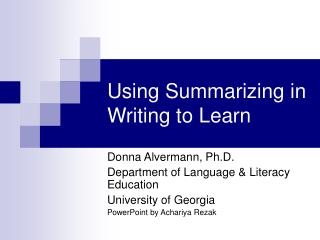Download PresentationUsing Summarizing in Writing to Learn

# Using Summarizing in Writing to Learn - PowerPoint PPT PresentationDownload Presentation## Using Summarizing in Writing to Learn

- - - - - - - - - - - - - - - - - - - - - - - - - - - E N D - - - - - - - - - - - - - - - - - - - - - - - - - - -
##### Presentation Transcript

1. Using Summarizing in Writing to Learn Donna Alvermann, Ph.D. Department of Language & Literacy Education University of Georgia PowerPoint by Achariya Rezak

2. What is the purpose of teaching summarizing? • Writing summaries is an important tool for comprehension because summarizing reinforces and consolidates many processes involved in learning from a text. • Writing a good summary means that readers must know how to select and delete information, condense information, and transform it into writing.

3. Three commonly used summary strategies are: • Hierarchical summaries: best used with text that is structured into headings and subheadings. • REAP: Read text. Encode it in your own words. Annotate and write it down. Ponder what you wrote. • GIST: Generating Interactions between Schemata and Text.

4. 1. As a class, scan the reading to preview headings and highlighted vocabulary. 2. Together, develop a skeleton outline based on these text clues. 3. Then, read text using the outline as a reading guide. 4. Ask each student to compose main idea statements for the main points, adding details. 5. Finally, develop a key idea statement for the entire passage. This becomes the first sentence of the summary. Implementing hierarchical summaries:

5. REAP: • Acronym that stands for: Read text. Encode it in your own words. Annotate and write it down. Ponder the message. • REAP can be any sort of annotation, from critical comments to personal reactions. • Summary annotations are the easiest.

6. Implementing REAP: • As a class, show students a sample paragraph and annotation. Explain the annotation process that you went through. • Next, show three annotations of a text and let students choose the best of the three. • Finally, show students how to develop summaries from their own annotations. • After that, students can develop their own summary annotations and then analyze these summaries in small groups to develop a more complete summary.

7. GIST: • Acronym: Generating Interactions between Schemata and Text. • Used to produce progressively more condensed summaries of a text

8. Implementing GIST: • As a class, use a short, coherent expository paragraph, and ask students to retell the first sentence in 15 words or less. • Add the second sentence, and have students summarize the first and second sentences together in 15 words or less. • Continue until the entire paragraph is summarized in 15 words or less. • Then, have the class summarize an entire paragraph at one time. • Finally, students try the strategy on their own.

9. Example of GIST: Paragraph: Students’ Conceptions of a Mathematical Definition (Zaslavsky & Shir, 2005) The notion of a mathematical definition develops over the schooling years mostly through examples of definitions of many specific mathematical concepts. Students rarely discuss nonexamples of a mathematical definition, nor do they deal explicitly with the imperative features of a definition, let alone come across a formal definition of the notion of a mathematical definition. In this sense, the concept of definition is to some extent similar to the concept of proof. However, although many studies address students’ or teachers’ conceptions of proof (e.g., Fischbein, 1982; Hoyles, 1997; Knuth, 2002), few studies investigate students’ conceptions of a mathematical definition (p. 318). • Summary of first sentence: Students develop ideas about mathematical definitions through examples from school classes. • Summary of first two sentences: Students develop ideas about mathematical definitions from school, but do not encounter a formal definition, or “nonexamples”. • Summary of first three sentences: Students develop ideas about mathematical definitions from school that are similar to the concept of the proof, but do not encounter a formal definition.

10. Helpful hints: • Self-contained passages of expository text with explicitly stated main ideas and a clear structure are easiest to work with. • The main ideas of a text aren't always obvious: point out that summaries should capture ideas that would be important to the author.

11. Summary: • GIST, REAP and hierarchical summaries are three strategies used to summarize texts. • These strategies will help reinforce and consolidate many comprehension processes involved in learning from text.

12. Reference • Zaslavsky, O. and Shir, K. (2005). Students’ conceptions of a mathematical definition. Journal for Research in Mathematics Education, 36/4, 317-346.